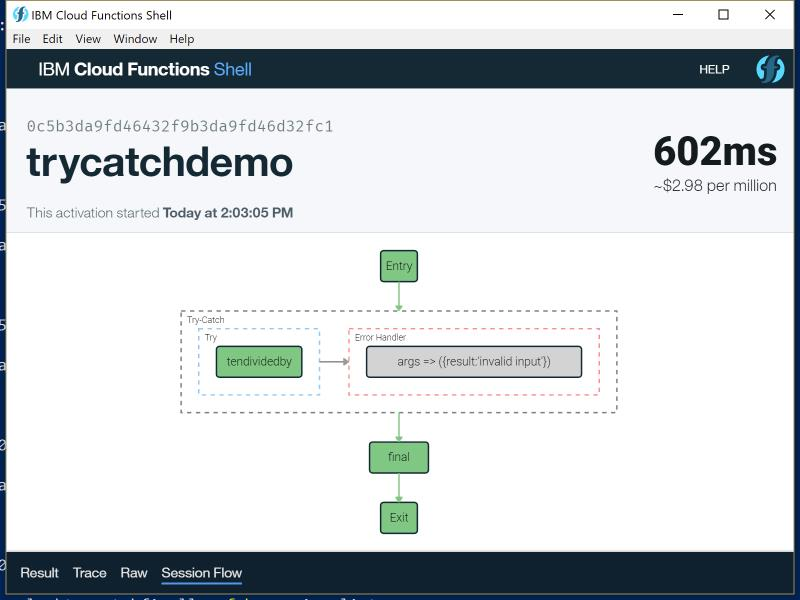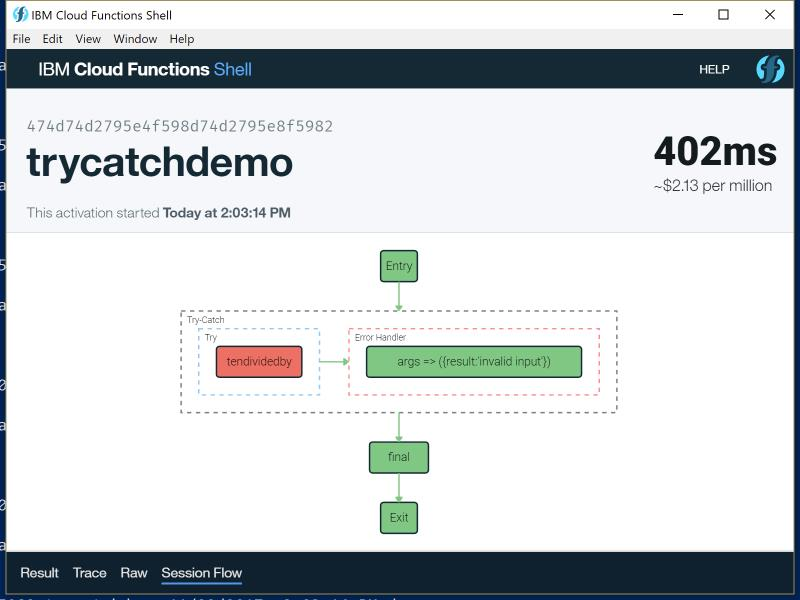It's been a few weeks since I blogged about IBM Composer, sorry about that, flying to China and getting a kid will put a kink into your blogging schedule. ;) Today I want to share a simple demo of how to wrap serverless functions with try/catch and try/catch/finally logic. Let's start off with a simple function.

``````
function main(args) {

if(!args.input) args.input = 0;

if(args.input === 0) {
throw new Error("Can't divide by zero and maintain the Universe.");
}

return { result: 10/args.input };

}
``````

This function simply takes an input value and then returns the result of dividing it into ten. If the input is 0, an error is thrown. I called this function `tendividedby`. Here's a sample result using an input of 2:

``````{
"result": 5
}
``````

And here is a result when the input is 0:

``````{
"error": "An error has occurred: Error: Can't divide by zero and maintain the Universe."
}
``````

If you get the activation record for the last test, you can clearly see it reported as an error:

``````"response": {
"status": "action developer error",
"statusCode": 0,
"success": false,
"result": {
"error": "An error has occurred: Error: Can't divide by zero and maintain the Universe."
}
},
``````

Alright, so what if we don't want an error reported? This is where a try/catch composition will come in handy. I begin by creating a new file for my composition, app.js. Here's the code.

``````composer.try(
'safeToDelete/tendividedby',
args => ({result:'invalid input'})
);
``````

The composition consists of one command, `composer.try`. This command takes two arguments - the action to try running and an action to run on failure. In the example above an inline action is being used but you can definitely pass the name of an existing action instead. My inline action simply says to return a result with a string indicating the error. You could do other things of course, for example sending an email about the error so people could be notified.

So that's try/catch, nice and simple. How about try/catch/finally? While this isn't built into the composer function itself, you can "fake" it by simply using a sequence where the "finally" part comes after the try/catch. Here's how that could look:

``````composer.sequence(
composer.try(
'safeToDelete/tendividedby',
args => ({result:'invalid input'})
),
'safeToDelete/final'
)
``````

In this case, I've set the "finally" part of my logic to be an action called final. All that does is add a timestamp:

``````function main(args) {
//just take the result and add a time
args.timestamp = new Date();

return args;
}
``````

In case you're curious, this could also be an inline function, but I wanted to demonstrate a mixture of both. To start working with this composition, first I create it:

fsh app create trycatchdemo app.js

Then I invoke it:

fsh app invoke trycatchdemo --param input 5

This results in:

``````{
result: 2,
timestamp: "2017-11-22T13:52:32.177Z"
}
``````

And here is the error condition version:

``````{
result: "invalid input",
timestamp: "2017-11-22T13:52:53.489Z"
}
``````

What's cool is that the Composer's graphical shell does a kick butt job of rendering these calls. So for example, here is the good test:And here is the bad run:If you have any questions about these examples, let me know!